# Simulation of Heat Transfer in Different Geometries Immersed in a Solar Pond Using Fortran and COMSOL Codes

Simulation of Heat Transfer in Different Geometries Immersed in a Solar Pond Using Fortran and COMSOL Codes
Laboratoire de l’Ingénierie des Procédés de l’Environnement (LIPE), Université Constantine 3, Constantine 25000, Algeria

Corresponding Author Email:
samah.zermane@univ-constantine3.dz
Page:
231-239
|
DOI:
https://doi.org/10.18280/ijht.380125
12 August 2019
|
Accepted:
8 November 2019
|
Published:
31 March 2020
| Citation

OPEN ACCESS

Abstract:

In this paper, we have numerically studied the phenomenon of unidimensional convection-conduction equation in a channel, cylinder and sphere immersed in a solar pond. Then we have studied bidimensional case in a heat exchanger, which undergoes an external heat flow provided by solar pond.

We carried out the discretization of the mathematical model by finite differences method (explicit scheme). Calculation codes in Fortran language have been created for the temperature distribution in all these geometries.

We presented the numerical results in terms of temperature profile functions, one- and two-dimensional, in laminar flow regime, for various parameters.

We also present a study by COMSOL of the same phenomenon on these geometries. This study can be presented by two methods, the first using the physical model and the second using the mathematical part of COMSOL.

Keywords:

convection conduction, finite differences method, solar pond, COMSOL

1. Introduction

Simulations now play an important role in scientiﬁc and engineering sciences, since they allow for faster and cheaper prototyping compared, e.g., to experiments. However, in many applications the overall goal is not just to simulate a given process or problem, but to obtain an optimal solution, optimal parameters or an optimal design or geometry .

A heat transfer within a system only occurs if there are temperature gradients between the different parts of the system, which implies that the system is not in thermodynamic equilibrium. During the transformation of the system to a state of final equilibrium, the temperature will change both in time and space. The purpose of analyzing heat transfer is to identify which transfer modes are involved during the transformation and to quantitatively determine how the temperature at each point of the system changes over time.

Thermodynamics show us that energy can be transferred from interactions between the system and its environment, in the form of heat and operation.

However, thermodynamics is only concerned with the initial and final state of the system to achieve equilibrium, and provides no information about the type of the interactions involved and about the temporal evolution of the system between the two equilibrium states [2, 3].

The convection-diffusion equation is a combination of both diffusion and convection equations. It describes physical phenomena where particles, energy or other physical quantities are transferred within a physical system due to two processes: diffusion and convection.

It has been used to describe heat transfer in a draining film; water transfer in the soil, dispersion of tracers in porous media, dispersion of contaminants in shallow lakes, propagation of solute in a liquid circulating in a tube, long-range transport of pollutants in the atmosphere, and dispersion of dissolved salts in groundwater [4, 5].

There are several areas where the advection-diffusion equation is applied, we give as an example:

In biology, the reaction - diffusion - advection equation is used to model the chemotaxis observed in bacteria, the migration of populations, evolutionary adaptation to changes in environments and the Spatio-temporal dynamics of molecular species, including morphogenesis 

In semiconductor physics, when carriers are generated (electrons and holes) due to the light shining in the center of an intrinsic semiconductor, they diffuse to both ends. The diffusion advection equation is used to study this phenomenon, where the electron diffusion constant is higher than that of the holes, which reduces the number of excess electrons in the center compared to the holes .

In medicine, the injection of a product takes a total time t or the blood in the vein flows with medium speed u; the concentration of chemical in the blood is C0, the equation studied gives the distribution of the chemical in the vein when it reaches the heart several seconds later .

In the Process Engineering industry, heat exchangers equipped with an external heating system given by a solar pond, the equation studied gives the temperature distribution of the solid and liquid along the exchanger at any time .

Among the authors who have conducted work on convection-conduction are:

Azzi  and Sbai , who presented two works, in which they solved the equation of convection - conduction by finite volumes.

Imakhlaf  suggested to estimate the heat flow through the outer wall of a pipe by the reverse method, involving heat exchange by forced convection.

Mojtabi  solved the convection-conduction equation by finite elements.

de Assis and Romao , worked on that phenomenon in pipes and spheres by discretizing the advection-diffusion equations through finite differences, using Crank Nickolson's scheme.

Several authors have presented the resolution of this equation using different methods [15-18].

In heat exchanger applications, it is often necessary to analyze the way in which heat conduction in solid components interacts with the convection processes in the fluid medium, with the objective of optimization of design or performance. The context of this analysis may be the study of extended surface design (fins) or the design of laminar flow heat exchangers, where heat conduction along separating walls may have a significant effect on heat exchanger performance .

Several researchers have worked on the nuts in heat exchangers, among them we can cite:

Renze and Akermann  proposed a study on the convective heat transfer characteristics in a periodic corrugated channel. It is investigated experimentally and numerically using the Finite Volume Method (FVM). Experimental results are used for the validation of the numerical results. In the numerical studies, Reynolds Averaged Navier-Stokes (RANS) based turbulence models are used. The turbulence models are compared with each other. Studies were conducted for air flow conditions where contact angle is 30°.

Rutgers  proposed work concerns the study of Numerical analysis on the fluid flow and heat transfer in corrugated channel with various geometry configurations, under constant heat flux conditions is considered. This is largely studied both experimentally and numerically due to their wide industrial application in various fields such as nuclear reactor urgency, solar boiler, heat exchangers and thermo siphon solar capturers, etc.

Vollaro et al.  proposed a study on convection. The objectives of this work are to study the hydrodynamic and thermal behaviour of cold and hot fluids used in double pipe heat exchanger, and compare the actual heat transfer observed by number of transfer unit method. Yet another objective is to simulate the obtained results using the CFD. In this approach, a model developed by computational fluid dynamics has been validated by actual heat transfer. The results from the numerical simulation of actual heat transfer well agreed with the effectiveness of heat transfer obtained from NTU method. The actual heat transfer (Q) acquired by CFD simulation and the mathematical NTU method were respectively, 29.7 KW and 30.5 KW. It clears that the percent error between the approaches, CFD simulation and effectiveness of heat transfer NTU method is 2.6%. It is less than the standard error (15%) and well acceptable.

Aslan et al.  proposed a study on the external convection at pipes and internal pipe heat transfer were investigated. The validity of the techniques was shown for each test case by comparing the simulation results with experimental and analytic data available in the literature. Finally, a simplified shell-and-tube heat exchanger was simulated to demonstrate how these methods can be applied to plant scale engineering problems.

Rehmani et al.  present Two new models for the heat exchanger were built based on a discretized finite volume method. Both flows and the wall were divided in elements and the laws for mass and energy conservation were applied. The heat capacitance of the wall was included in the calculations as well. The first model is based on iterative calculations of the parameters, while the second model uses the values of the previous time step to calculate the temperature, enthalpy and heat exchange.

Debtera et al.  proposed a study on A microclimatic analysis in a typical urban configuration, has been carried out using Ansys Fluent Version 14.0, an urban street canyon, with a given H/W ratio, has been considered to simulate a three-dimensional flow field and to calculate the thermal fluid dynamics parameters that characterize the street canyon. In this paper, the convective heat transfer coefficient values on the windward external façade of the canyon and on the windward and leeward inner walls are analyzed and a comparison with values from experimental and numerical correlations is carried out.

2. Description of the Physical Phenomenon

2.1 Unidimensional flow

The Figure 1 shows a heat system in a solar pond of length L, height d, and wall epessor E, such as (d<<L), crossed by a fluid flow that enters with an inlet velocity Ue and a temperature Te. We will study the temperature distribution in this system according to position and time in different cases.

## 1.png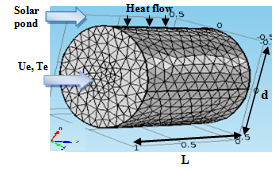Figure 1. Description of the geometric model

We consider the equation of the transport of a physical entity in the cartesian, cylindrical and spherical coordinates.

$\frac{\partial T}{\partial t}+u x \frac{\partial T}{\partial x}=D \frac{\partial^{2} T}{\partial x^{2}}+S$       (1)

$\frac{\partial T}{\partial t}=\left(\frac{D}{r}-u_{r}\right) \frac{\partial T}{\partial r}+D \frac{\partial^{2} T}{\partial r^{2}}+s$      (2)

$\frac{\partial T}{\partial t}=\left(\frac{2 D}{r}-u_{r}\right) \frac{\partial T}{\partial r}+D \frac{\partial^{2} T}{\partial r^{2}}+S$      (3)

These geometries have an extern energy given by the solar pond. Its present external heat flow:

$Q=h . A .(T f e-T)$

Initial Condition

$\mathrm{t}=0, \mathrm{I}(\mathrm{x}, 0)=\mathrm{f}(\mathrm{x})=4 x(1-\mathrm{x})$

Conditions to the limits

At:     x = 0, T= 0, x = L, T(L,t) = 0

2.2 Bidimensional flow

In the following, we present a case of a heat exchanger that undergoes an external heat flow of several configurations. A fluid enters the exchanger with a constant speed and temperature. The heat transfer is done on both sides, from the fluid and solid part.

The mathematical formulation of this heat transfer problem, covering the areas of solids and fluids, can be expressed as follows:

Fluid Part:

$\begin{array}{c} \frac{\partial T_{f}}{\partial t}+u_{x} \frac{\partial T_{f}}{\partial x}=D_{f}\left(\frac{\partial^{2} T_{f}}{\partial r^{2}}+\frac{1}{r} \frac{\partial T_{f}}{\partial r}+\frac{\partial^{2} T_{f}}{\partial x^{2}}\right) \\ u_{x}=2 u_{\operatorname{moy}}\left[1-\left(\frac{r}{R}\right)^{2}\right] \end{array}$        (4)

Solid Part:

$\frac{\partial T_{s}}{\partial t}=D s\left(\frac{\partial^{2} T_{s}}{\partial r^{2}}+\frac{1}{r} \frac{\partial T s}{\partial r}+\frac{\partial^{2} T_{s}}{\partial x^{2}}\right)$      (5)

For the scaling of the system of equations, we consider the following quantities:

$\hat{\mathrm{r}}=\frac{\mathrm{r}}{\mathrm{R}}, \tau=\frac{\mathrm{D}_{\mathrm{f}} \mathrm{t}}{\mathrm{R}^{2}}$$\theta=\frac{\mathrm{K}\left(\mathrm{T}-\mathrm{T}_{0}\right)}{\mathrm{Rq}_{\mathrm{réf}}}$$\mathrm{U}=\frac{\mathrm{U}_{\mathrm{x}}}{\mathrm{U}_{\mathrm{moy}}}, \mathrm{A}=\frac{\mathrm{D}_{\mathrm{s}}}{\mathrm{D}_{\mathrm{f}}}$

Fluid Part:

$\frac{\partial \theta_{\mathrm{f}}}{\partial \tau}+\operatorname{RePr} \frac{\mathrm{R}}{\mathrm{L}} \frac{\partial \theta_{\mathrm{f}}}{\partial \mathrm{X}}=\left[\frac{\partial^{2} \theta_{\mathrm{f}}}{\partial \hat{\mathrm{r}}^{2}}+\frac{1}{\hat{\mathrm{r}}} \frac{\partial \theta_{\mathrm{f}}}{\partial \hat{\mathrm{r}}}+\left(\frac{\mathrm{R}}{\mathrm{L}}\right)^{2} \frac{\partial^{2} \theta_{\mathrm{f}}}{\partial \mathrm{X}^{2}}\right]$            (6)

Solid Part:

$\frac{\partial \theta_{s}}{\partial T}=A\left[\frac{\partial^{2} \theta_{s}}{\partial \hat{r}^{2}}+\frac{1}{\hat{r}} \frac{\partial \theta_{s}}{\partial \hat{r}}+\left(\frac{R}{L}\right)^{2} \frac{\partial^{2} \theta_{s}}{\partial X^{2}}\right]$     (7)

Initial and boundary conditions:

$\begin{array}{c} \tau=0 ; \theta_{s}=\theta_{\mathrm{f}}=0 \\ \mathrm{X}=0 ; \theta_{\mathrm{s}}=\theta_{\mathrm{f}}=0 \\ \mathrm{X}=1 \text { et } 0<\hat{\mathrm{r}}<1+\mathrm{E} ; \frac{\partial \theta_{\mathrm{s}}}{\partial \mathrm{x}}=\frac{\partial \theta_{\mathrm{f}}}{\partial \mathrm{x}} \\ \hat{\mathrm{r}}=0 \text { et } 0<\mathrm{X}<1 \frac{\partial \theta_{\mathrm{f}}}{\partial \hat{\mathrm{r}}}=0 \\ \hat{\mathrm{r}}=1 \text { et } 0<\mathrm{X}<1 \theta_{\mathrm{s}}=\theta_{\mathrm{f}} \\ \hat{\mathrm{r}}=1 \text { et } 0<\mathrm{X}<1 ; \mathrm{k}_{\mathrm{s}} \frac{\partial \theta_{\mathrm{s}}}{\partial \hat{\mathrm{r}}}=\mathrm{k}_{\mathrm{f}} \frac{\partial \theta_{\mathrm{f}}}{\partial \hat{\mathrm{r}}} \\ \hat{\mathrm{r}}=1+\mathrm{E} \text { et } 0<\mathrm{X}<1 ;-\frac{k_{s}}{k_{f}} \frac{\partial \theta_{s}}{\partial \hat{\mathrm{r}}}=\mathrm{Q} \end{array}$

3. Method of Resolution

We carried out the discretization of the mathematical model using the numerical method of finite differences (explicit scheme). Calculation codes in Fortran language have been created for temperature distribution in a channel, cylinder and sphere. The general flow chart is presented to solve the conduction-convection problem where the stability condition of the explicit scheme is respected: l= (D.Dt/Dx2) ≤ ½ .

4. Results and Discussion

4.1 Validation of calculated code

To validate the Fortran code, we compared the resolution of the diffusion equation by finite differences and separation of variables, the latter was developed to find the following final equation, these results is confirmed by Christelle et al. [26-28]:

T(x,t) = g(x). f(t)

After a demonstration we find:

$\mathrm{T}(\mathrm{x}, \mathrm{t})=\mathrm{Cn} \cdot \sin \left(\frac{\pi \cdot \mathrm{n}}{\mathrm{L}} x\right) B n \cdot e^{\left(-D\left(\frac{\pi \cdot \mathrm{n}}{\mathrm{L}}\right)^{2} t\right)}$       (8）

$T(x, t)=\sum_{n=1}^{\infty}\left[A_{n} \sin \left(\frac{\pi \cdot \mathrm{n}}{\mathrm{L}} x\right) e^{\left(-D\left(\frac{\pi \cdot \mathrm{n}}{\mathrm{L}}\right)^{2} t\right)}\right]$        (9)

With: An = Cn. Bn

In our case:

$T(x, t)=f(x)=\sum_{n=1}^{\infty}\left[A_{n} \sin \left(\frac{\pi \cdot \mathrm{n}}{\mathrm{L}} x\right)\right]$

And:

$A n=\frac{2}{L} \int_{0}^{L} f(x) \cdot \sin \left(\frac{\pi \cdot n}{L} x\right) d x$

So, we will finally:

\begin{aligned} T(x, t) &=\sum_{n=1}^{\infty}\left[\left(\frac{2}{L}\right) \int_{0}^{L} f(x) \sin \left(\frac{\pi \cdot n}{L} x\right)\right.\\ d x &\left.\left.\sin \left(\frac{\pi \cdot n}{L} x\right) e^{\left(-D\left(\frac{\pi \cdot n}{L}\right)^{2} t\right)}\right)\right] \end{aligned}       (10)

We programmed this equation by calculating the integral by the trapezoid method.

The Figure 2 represents the temperature distribution along a full channel, the diffusion equation is solved by two methods: separation of the variables, and finite differences:

## 2.png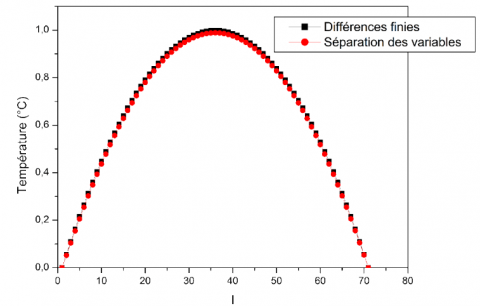Figure 2. Validation of calculated code

From the calculation of the maximum error using the formula (11), we find that the maximum error is equal to 10.52% which is very acceptable for the validation of our program and therefore our code is correct and we will modify it to solve the following problem.

$\text {Error }(\%)=\frac{\left(T_{\text {diff}}-T_{\text {sep}}\right)}{T_{\text {diff}}} * 100$     (11)

4.2 Results of unidimesional flow with fortran

4.2.1 Spatio-temporal representation

In the following section (Figure 3), we will study the results of the convection-diffusion problem in a different system where a fluid enters with a constant temperature and velocity, and with an initial triangular shape condition: f(r).

## 3a.png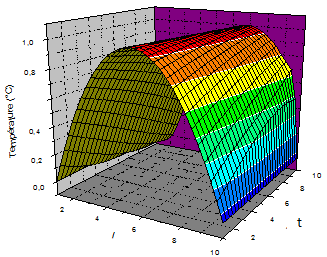(a) channel

## 3b.png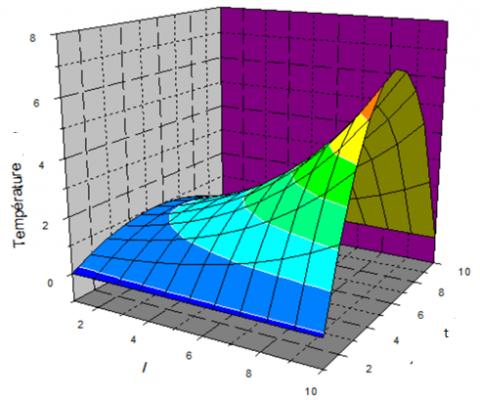(b) cylinder

## 3c.png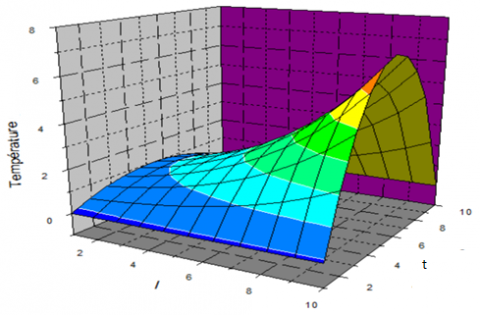(c) sphere

Figure 3. Spatio-temporal representation

Several assumptions are kept for the study of a one-dimensional case such as:

-Good material conductivity,

-Length much greater than transversal dimensions

-Consistent external fluid temperature.

This is done by studying the effect of several variables.

The temperature distribution of the Newtonian and incompressible fluid in our systems is represented by a Spatio-temporal configuration.

The objective of this presentation is to study the mechanisms and kinetics of fluid heat exchange.

According to the study of the mono-dimensional temperature field T(r,t) where we were able to obtain the temperature at each point of our medium, we notice its increase as a function of position until reaching a maximum value, then decreases in a parabolic way. This is due to the influence of initial conditions and to the problem's limits. The same remark is made over time, but with higher values in relation to temperatures. This is due to the evolution of heat transfer over time, until reaching an established scheme where there will no longer be a heat transfer starting from (10 s).

4.2.2 Influence of initial conditions

The Figure 4 shows the temperature profiles for several initial conditions under the effect of two different equations of initial conditions:

## 4a.png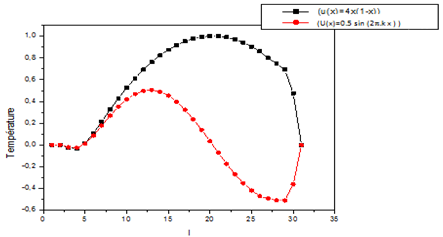(a)

## 4b.png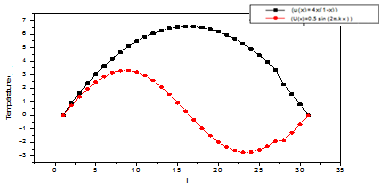(b)

## 4c.png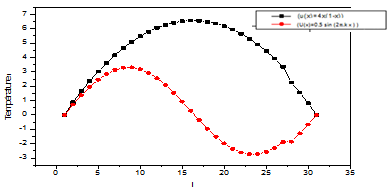(c)

Figure 4. Influence of initial conditions on profiles of temperature (a) channel, (b) cylinder and (c) sphere

1) By applying the first equation (at t=0, T(x,0) = 4x(1-x)), we found that at the beginning, the temperature is stable then increases quickly to reach a high value, then decreases by presenting a sort of wave.

2) With regard to the second equation which is in the form of a sinusoidal function (at t=0, T(x,0)= 0.5sin(2pkx)) we notice the same remark, but there is a difference in the last part, where the temperature drops to negative values and then increases while presenting a faster wave.

Both conditions are of Dirichlet type, where the system temperature is initially imposed resulting in a convection-conduction transfer.

This is due to the type of the equation itself, and so it is claimed that the initial conditions play a very important role in the spatial distribution of temperature.

4.3 Results of bidimensional flow with Fortran

One of the essential elements of an energy system is the heat exchanger, which is involved in almost all heat exchange processes.

A large part of the thermal energy used in industrial processes passes through a heat exchanger at least once.

Let’s consider a heat exchanger (of length L, radius R, and thickness T), assuming that losses are eliminated by thermal insulation of the lateral surface, having two configurations:

• First configuration:

First configuration presented in Figure 5 has a sinusoidal evolution function, which is a heat flow that varies with time and position: Q (x,t )=20x sin(pt).

## 5.png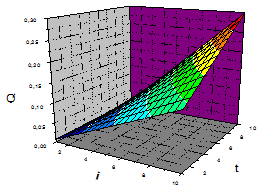Figure 5. First imposed heat flow

Spatio-temporal representation for first configuration is shown in Figure 6.

## 6a.png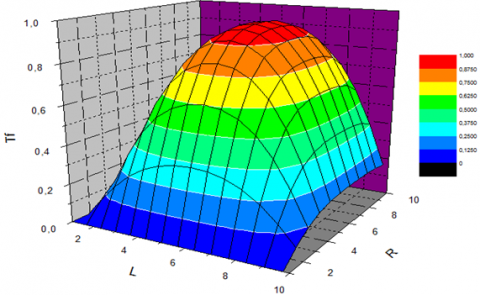(a)

## 6b.png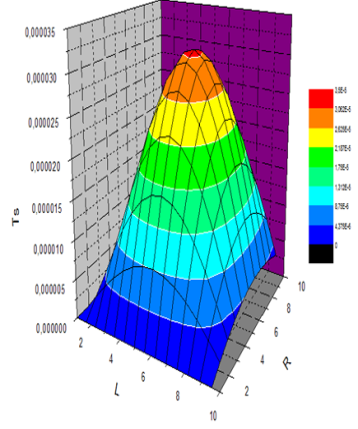(b)

Figure 6. Spatio-temporal representation for first configuration. (a) Fluid temperature (b) solid temperature

• Second configuration

Second configuration (Figure 7) has a Triangular evolution such as:

Q (t)= 20t if t ≤ 1000 and Q (t) = 20(1-t) if t> 1000

## 7.png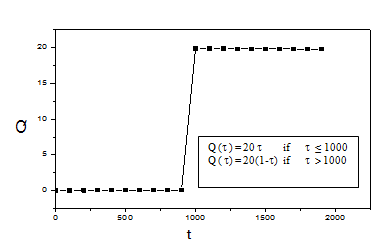Figure 7. Second imposed heat flow

Spatio-temporal representation for second configuration is shown in Figure 8.

The temperature results as a function of time and both dimensions are dimensionless. The use of this form is more favorable because it limits the number of parameters and presents the results in a general form.

There is a very noticeable heat transfer from the outer wall particularly from the part where the heat flow is provided, towards the fluid which makes the latter heated.

This convection-conduction phenomenon causes the temperature of the fluid and solid to change in a parabolic manner along the exchanger and the radius, but more localized in the case of the solid.  On the other hand, the objective of calculating the distribution of solids temperatures is to ensure that the temperature of the materials remains within reasonable limits dictated by mechanical properties and corrosion resistance, and also to avoid thermal stresses. The temperature values of solids decrease over time, which implies the transfer of temperature from the solid part to the fluid part.

Thus, the flow is conducted from the outer wall to the inner wall of the pipe where heat is transferred to fluid in a combined manner (conduction and convection).

## 8.png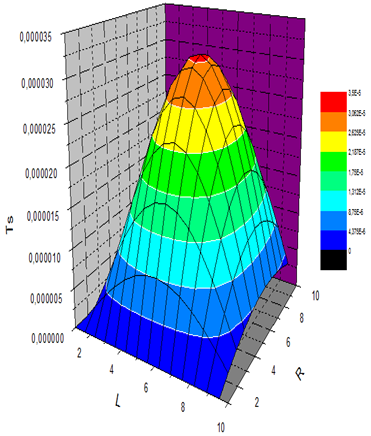Figure 8. Spatio-temporal representation for second configuration. Fluid temperature (left) solid temperature

• Influence of Reynolds number

For the same heat exchanger with an external flow of two configurations, the influence of the Reynolds number on the temperature distribution was studied:

In Figure 9, We clearly notice that the Reynolds number does not have an influence on the thermal transfer with regard to the first configuration, unlike the second case where we find a temperature evolution according to the Reynolds number.

## 9.png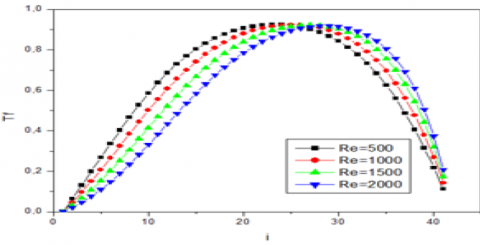Figure 9. Influence of Reynolds number on fluid profile temperate

This is explained by the predominance of conduction in the first case where the conductive flux is distributed according to a sinusoidal function as a function of position and time, and by the predominance of convection in the second case where the conductive flux is distributed according to a triangular function as a function of time.

5. Solving of the Advection-Diffusion Problem with COMSOL

COMSOL Multiphysics is a cross-platform finite element analysis, solver and multiphysics simulation software. It allows conventional physics-based user interfaces and coupled systems of partial differential equations (PDEs). COMSOL provides an IDE and unified workflow for electrical, mechanical, fluid, acoustics and chemical applications [29, 30].

COMSOL presents two methods for solving the diffusion convection equation.

• Multiphisics COMSOL

Under part heat transfer we will choose the transfer in fluids and solids in laminar regime.

• COMSOL mathematical functions

Under mathematical part, we find classical differential equations where we will choose the convection conduction type equation, or our study will be temporal. In these two cases we will choose our geometry, the suitable materials (water circulating inside and aluminum as solid material of the walls), the choice of the initial and boundary conditions is essential, we will complete our study by the choice of mesh (Figure 10) presented in bidimensional coordinates

The following Table 1 presents a comparison of the temperatures of the different geometries studied after simulation

## 10.png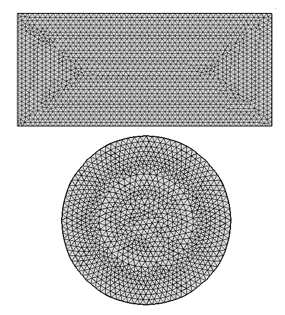Figure 10. Mesh of studied geometries given by COMSOL

Table 1. Comparison between the few results of the Calandar and circular systems

 Configuration Temperature (K) at 0.25 cm 5 seconds 20 seconds Calandar system Circular system 150 100 70 65

5.1 Results of unidimensional flow with COMSOL

The results obtained for the same geometries by COMSOL are shown in the Figure 11 and 12:

The Conjugate Heat Transfer, Laminar Flow multiphysics interface is used to simulate the coupling between heat transfer and fluid flow.

## 11.png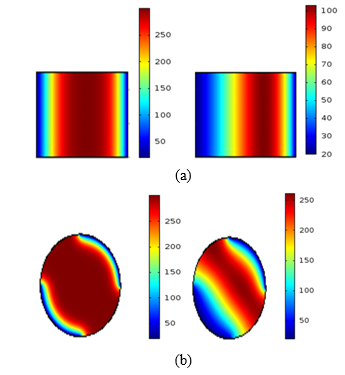Figure 11. Temperature airs in calandar and circulal systems by COMSOL (a) t=5 seconds (b) t=20 seconds

## 12.png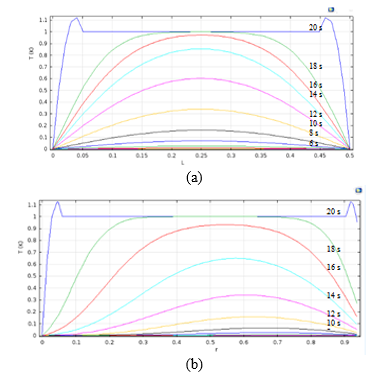Figure 12. Profiles of Temperature in (a) calandar and (b) circulal systems by COMSOL

It combines the Heat Transfer in Solids and Laminar Flow interfaces. The Non-Isothermal Flow multiphysics coupling is automatically added.

5.2 Results of bidimensional flow with COMSOL

The Figures 13 show the distribution of temperature, speed, and pressure inside the heat exchanger.

## a.png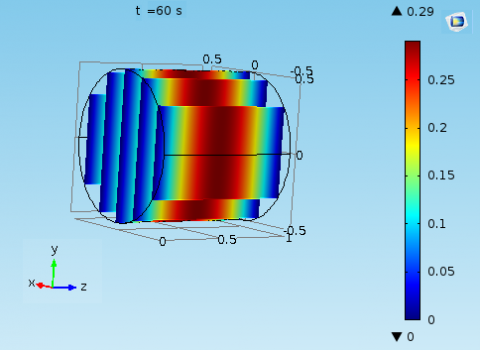(a)

## b.png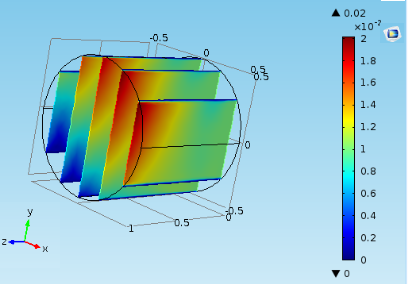(b)

## c.png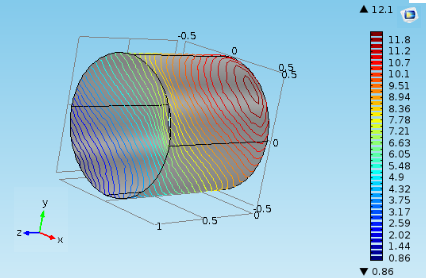(c)

Figure 13. Tridimensional representation of (a) temperature (b) velocity (c) pressure in a heat exchanger

The fluid which flows along an exchanger placed in the direction of flow. The speed of the fluid is zero at the wall, and, due to friction, increases progressively through the fluid when one moves away from the wall until reaching the speed far from the wall. This effect is considered to be confined in a thickness of fluid called the boundary layer, close to the solid wall.

Viscosity generates a speed gradient in a straight section of heat exchanger:

At the wall, the velocity of the fluid is zero (this is the adhesion condition); and is maximum at the center of the pipe. This generates heat transfer in the fluid.

The friction between the fluid and the walls leads to the dissipation of the mechanical energy of a fluid in the form of heat. This phenomenon is called pressure drop. This energy dissipation is commonly expressed in the form of a pressure variation

We note in Figure 14 the temperature profile along the exchanger as a function of time.

## 14.pngFigure 14. Profile of temperature in heat exchanger

The form of profile is parabolic due to the effects of speed and initial conditions and limits.

So, we could have by the energy storage of the solar pond have a free heating of the fluid which circulates inside the heat exchanger.

6. Conclusion

Heat transfer plays an important role in almost all industrial fields. This phenomenon is governed by partial differential equations whose solution requires the implementation of digital solutions that have now become indispensable.

The objective of this work is to solve a system of advection-diffusion equations and to study the effect of several parameters (boundary conditions) on the heat transfer temperature distribution in a channel, cylinder and sphere in a solar pond. A program, in Fortran language, has been elaborated, validated and adapted to address the problem highlighted in this work.

Results show that there are factors that influence the temperature distribution:

• A low flow velocity remains the most convenient for stable laminar transfer.
• A triangular initial condition is more convenient than a sinusoidal condition.
• The convection-conduction transfer remains more important in the middle of the channel and at the outlet of the cylinder and sphere.
• The transfer by convection-conduction remains more important during the time until the arrival at an established regime.
• Practically, there is not much difference between a transfer in a cylinder and sphere compared to the radial temperature distribution.

Concerning simulation with COMSOL we had by the use of the mathematical option to have the temperature fields in a cylindrical channel in a solar pond and shape in transient regime.

Nomenclature
 d Diameterm D Coefficient of diffusion m2/s h Heat convection coefficient W/m2K l Q t T Tfe Tf Ts Tfe U Re Pr Length m External heat flow W Times Temperature of internal fluid K Temperature of external fluid K Fluid temperature K Temperature of the solid K Temperature of solar pond K Flow velocity m/s Reynolds number Number of Prandtl Greek symbols τ Dimension less time $\theta f$ Dimension less temperature of the fluid $\theta_{S}$ Dimension less temperature of the solid $\alpha$ thermal diffusivity, m2.s-1 $\beta$ thermal expansion coefficient, K-1 $\phi$ solid volume fraction Ɵ dimensionlesstemperature µ dynamicviscosity, kg.m-1. s-1 Subscripts p nanoparticle f fluid(purewater) nf nanofluid
References

 Slawig, T., Prüfert, U. (2011). Mathematics-based optimization in the COMSOL multiphysics framework. Excerpt from the Proceedings of the 2011 COMSOL Conference in Stuttgart, pp. 1-6.

 Khider, F.Z. (2016). Mémoire de fin d'étude master, “thème: amélioration de la convection mixte en utilisant des ailettes cylindriques avec des ouvertures au niveau de la base.” faculté génie mécanique, universite m'sila.

 Marty, P. (2001). Cours de transferts thermiques. Université Joseph Fourier, Grenoble.

 Ahmed, G.S. (2012). A numerical algorithm for solving advection-diffusion equation with constant and variable coefficients. The Open Numerical Methods Journal, 4(1): 1-7.

 Sur, L. (2005). Influence de l’effet thermique sur les caracteristiques de la couche limite laminaire sur une paroi lisse. faculté génie mécanique,universite biskra. http://thesis.univ-biskra.dz/id/eprint/1137.

 Mesbahi, S. (2018). Analyse mathématique de systèmes de réaction-diffusion quasi-linéaires avec données non régulières. Doctoral dissertation.

 Haelterman, M. (1998). Physique des semi-conducteurs II. Université Libre de Bruxelles.

 Ursino, M. (2005). Modelling in medicine and biology VI. WIT Transactions on Biomedicine and Health, WIT Press, Vol. 8.

 Al Hadad, W., Jannot, Y., Maillet, D. (2015). Modélisation du transfert de chaleur dans un demi-échangeur. In Congrès Français de Thermique SFT 2015.

 Abbés, A.Z.Z.I. (2003). Méthodes numériques appliquées au calcul des écoulements et du transfert de chaleur. USTO MB. Université de Oran.

 Sbai, A. (2016). Méthode des volumes finis pour l’équation de convection-diffusion memoire de fin d’etudes pour l’obtention du diplôme de master sciences et techniques, faculte des sciences et techniques fes – saiss.

 Imekhlef, A. (2013). Analyse d’un problème inverse de conduction-convection forcée dans un échangeur de chaleur, memoire présenté à la faculté des sciences département de physique pour l’obtention du diplôme de magister, option: energie renouvelables, universite Setif-1.

 Mojtabi, A., Deville, M.O. (2015). One-dimensional linear advection–diffusion equation: Analytical and finite element solutions. Computers & Fluids, 107: 189-195. https://doi.org/10.1016/j.compfluid.2014.11.006

 de Assis, L.H.P., Romao, E.C. (2018). Numerical simulation of 1D unsteady heat conduction-convection in spherical and cylindrical coordinates by fourth-order FDM. Engineering, Technology & Applied Science Research, 8(1): 2389-2392.

 Hasson, M.T. (1983). Résolution de l'équation de convection-diffusion et d'un modèle de circulations océaniques générales par des méthodes d'éléments finis, Doctoral dissertation.

 Medaouar Walid, Simulation numérique du phénomène d’advection et de diffusion de la concentration d’un polluant dans un écoulement permanent bidimensionnel par la méthode des volumes finis. projet de fin d’études en vue de l’obtention du diplôme de master université Hassiba Benbouali de chlef-2012.

 Dahmani, M., Akkouche, S., Seghir, A. (2018). Modélisation numérique du phénomène de transport de masse dans Les milieux poreux, Doctoral dissertation, Université Abderrahmane Mira-Bejaia.

 Bourassa, F. (2012). Résolution numérique de l’équation d’advection, centre escer.

 Davies, T.W. (2011). Coupled conduction and convection. A-to-Z guide to thermodynamics. Heat & Mass Transfer, and Fluids Engineering. http://dx.doi.org/10.1615/AtoZ.c.coupled_conduction_and_convection

 Renze, P., Akermann, K. (2019). Simulation of conjugate heat transfer in thermal processes with open source CFD. Chem Engineering, 3(2): 59. https://doi.org/10.3390/chemengineering3020059

 Rutgers, J. (2016). Internship at Powerspex Dynamic modeling of a heat exchanger University of Twente Faculty of Engineering Technology.

 Vollaro, A.D.L., Galli, G., Vallati, A. (2015). CFD analysis of convective heat transfer coefficient on external surfaces of buildings. Sustainability, 7(7): 9088-9099. https://doi.org/10.3390/su7079088

 Erman, A., Mardiros, E., Guven, O., Ilkay, C., Gokhan, K., Imdat, T., Yasar, I. (2015). CFD simulation for convection heat transfer in Corrugated channel. 8th ICCHMT, Istanbul, pp. 25-28. https://www.researchgate.net/publication/329556852

 Rahmani, K., Al-Kassir, A., Benalia, M., Djedid, M., Ad, C., Elmsellem, H., Steli, H., Kadmi, Y. (2017). Simulation of fluid flow and heat transfer inside a heat exchanger corrugated channel. JMES, 8(2): 566-572.

 Debtera, B., Neme, I., Prabhu, V. (2018). CFD simulation of a double pipe heat exchanger: Analysis conduction and convection heat transfer. International Journal of Scientific Research and Review, 7(12).

 Christelle, C. (2002). La méthode de séparation des variables au cycle 3. grand n, 79, à 100.

 Rondepierre, A., Rrouchon, A. (2013). iIntroduction aux équations aux dérivées partielles.

 Nelly point et Jacques-hervé Saiac, Equations aux dérivées partielles, mathématiques et méthodes numériques 2008.

 The COMSOL Group. COMSOL.com. COMSOL, Inc. Retrieved 22 June 2019.

 COMSOL Modeling Software. COMSOL.com. COMSOL, Inc. Retrieved 20 November 2015.